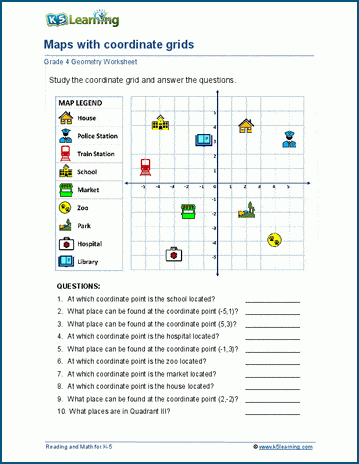# Coordinate Plane Worksheets

In grade 5 students start to learn about plotting points on coordinate grids, translating and rotating shapes on a coordinate plane, and plotting points on coordinate planes.

## What is a coordinate plane?

A coordinate plane is a two-dimensional surface formed by two intersecting lines: a horizontal X-axis and a vertical Y-axis. The point 0 at which they intersect is called the origin.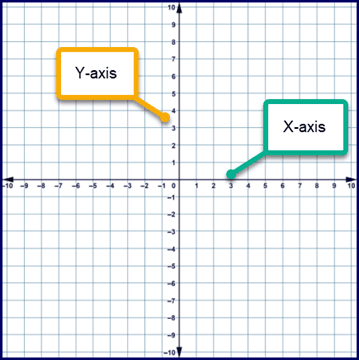## What are coordinates?

Coordinates are sets of two values that locate a specific point on a coordinate plane grid. That point is on the coordinate plane is named by its ordered pair: (x, y) – corresponding to the X-coordinate and the Y-coordinate. The coordinates can be positive, zero or negative, depending on the location of the point in the respective quadrants.

First quadrant: x > 0, y > 0

Second quadrant: x < 0, y > 0

Third quadrant: x < 0 , y < 0

Fourth quadrant: x > 0, y < 0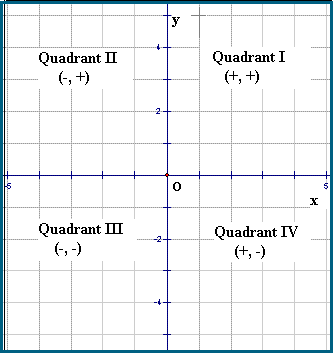We’ve created a number of new worksheet pages to cover the topic of plotting coordinates on a coordinate plane.

## Practice translations on a coordinate plane

In these worksheets, students translate and rotate simple shapes on a coordinate plane.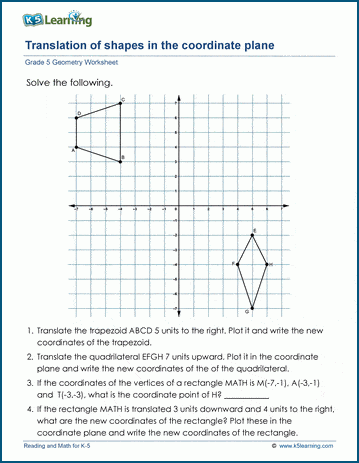## Function tables and the coordinate plane worksheets

Students are given a function, asked to work out the ordered pairs and plot the points on a coordinate grid.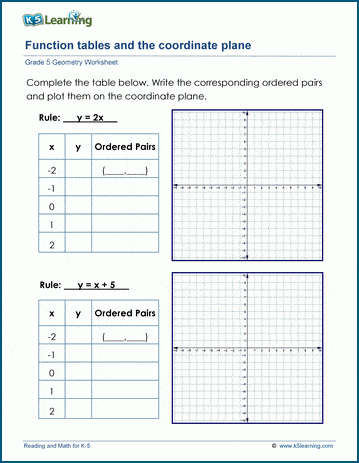## Discover the mystery picture by plotting coordinate grids

A fun spin on plotting points on a coordinate plane, these worksheets have students plot out mystery pictures.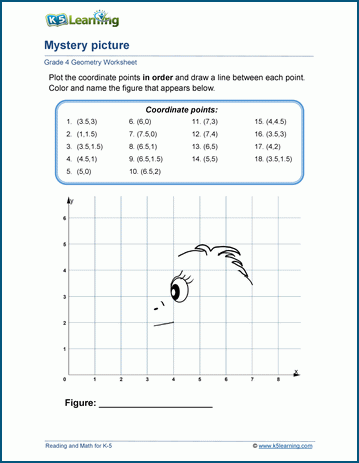## Discover the mystery message

Students discover a mystery message by locating clues in the coordinate plane. Another fun worksheet to help students learn about coordinates.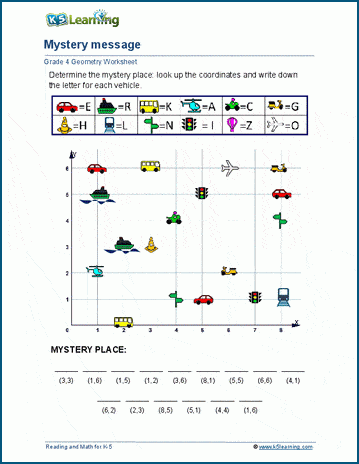## Study maps based on coordinate grids

Students study the points on a coordinate grid and the corresponding legend to answer a series of questions.